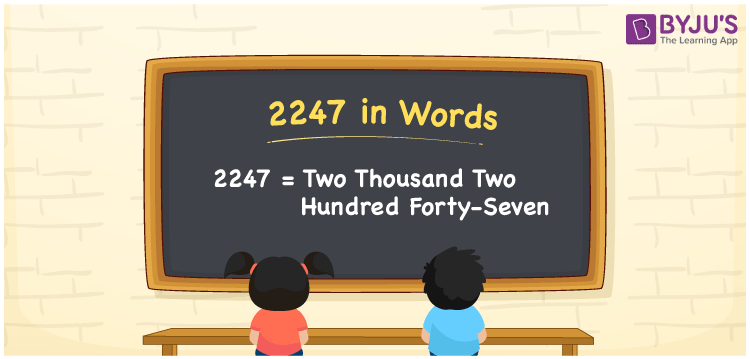# 2247 in Words

2247 in words can be written as Two Thousand Two Hundred Forty-Seven. If you buy one bag of rice for Rs. 2247, then you can say that “I bought a bag of rice for Two Thousand Two Hundred Forty-Seven Rupees”. Students will know the place value of the digits in 2247 using this article. Therefore, 2247 can be read as “Two Thousand Two Hundred Forty-Seven” in words.

 2247 in words Two Thousand Two Hundred Forty-Seven Two Thousand Two Hundred Forty-Seven in Numbers 2247

## 2247 in English Words## How to Write 2247 in Words?

In this section, you will be able to design the place value chart of 2247 on your own without any doubts.

 Thousands Hundreds Tens Ones 2 2 4 7

2247 in expanded form is:

2 x Thousand + 2 × Hundred + 4 × Ten + 7 × One

= 2 x 1000 + 2 × 100 + 4 × 10 + 7 × 1

= 2000 + 200 + 40 + 7

= 2247

= Two Thousand Two Hundred Forty-Seven

Therefore, 2247 in words is written as Two Thousand Two Hundred Forty-Seven.

2247 is a natural number that precedes 2248 and succeeds 2246.

2247 in words – Two Thousand Two Hundred Forty-Seven

Is 2247 an odd number? – Yes

Is 2247 an even number? – No

Is 2247 a perfect square number? – No

Is 2247 a perfect cube number? – No

Is 2247 a prime number? – No

Is 2247 a composite number? – Yes

## Frequently Asked Questions on 2247 in Words

Q1

### Write 2247 in words.

2247 can be written in words as “Two Thousand Two Hundred Forty-Seven”.
Q2

### How do you write Two Thousand Two Hundred Forty-Seven in numbers?

Two Thousand Two Hundred Forty-Seven can be written in numbers as 2247.
Q3

### Is 2247 an even number?

No, 2247 is not an even number as it is not completely divisible by 2.